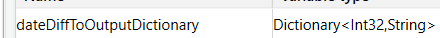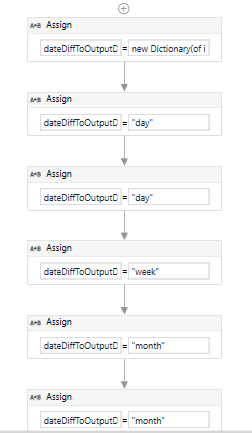# To decide the output based on date difference count

Hello All,

My query is , there are two inputs date 1 and date 2, now i am calculating datedifference between those two inputs and based on the output, bot need to decide whether its a day or week or month. Help me to get the output in step3!!!

Step1: getting input date 1 and date 2
Step2: Var_out = calculating datediff date 1 and date 2
Step 3: If Var_out is

1 or 3 which need to return output as “day”;
6 which need to return output as “week”;
29 or 30 which need to return output as “month”

assuming date1/date2 are string variables

1. get datediff (integer) using
`(DateTime.parse(date2) - DateTime.parse(date1)).TotalDays`

``````If datediff = 1 or datediff = 3
output = "day"

If datediff = 6
output = "week"

If datediff = 29 or datediff = 30
output = "month"
``````

@jack.chan -Is it possible by Without using if condition , how can i return the output by comparing datediff with 1,3,6,29,30

@Anusuya_R use dictionarythen do assign as follows:

``````dateDiffToOutputDictionary = new Dictionary(of int32, string)
dateDiffToOutputDictionary(1) = "day"
dateDiffToOutputDictionary(3) = "day"
dateDiffToOutputDictionary(6) = "week"
dateDiffToOutputDictionary(29) = "month"
dateDiffToOutputDictionary(30) = "month"
``````now, assign output as follows
`output = dateDiffToOutputDictionary(dateDiff).ToString`

this means
if datediff = 1, output = “day”
if datediff = 30, output = “month”
and so on…

Test (2).xaml (9.4 KB)

1 Like

This topic was automatically closed 3 days after the last reply. New replies are no longer allowed.